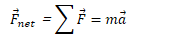# Newton's Second Law of Motion

• The acceleration of an object is in the direction of and directly proportional to the net force applied, and inversely proportional to the object's mass

• Valid only in inertial reference frames.

•# Mass vs. Weight

• Mass is the amount of "stuff" something is made up of.

• It remains constant

• Weight (mg) is the force of gravity on an object

• Weight varies with gravitational field strength (g)

# Newton's Third Law of Motion

• All forces come in pairs. If Object 1 exerts a force on Object 2, then the Object 2 must exert a force back on Object 1, which is equal in magnitude and opposite in direction

•